Domino Puzzles
 What is Domino? Domino Pieces Figures from Dominos Quadrilles Seven Squares Making Pairs  Dominos on the Internet  References.
 To the Main Page     "Mathematische Basteleien"

What is Domino?Domino is a famous game with domino pieces  (shorter dominos). Two or more players must alternately lay fitting pieces. The winner is the person who gets rid of all his pieces first.  Here you see a picture of the first phase of the play.
I won't go into details now. I shall describe puzzles with dominos and then only with all its 28 pieces.

Domino Pieces   top
You need eight sequences of seven squares for the pieces (down left). Altogether there are 56 squares. They show the numbers 0 to 6. The numbers are represented by patterns you find on dice. An empty square takes the place of the number 0.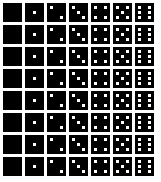Two squares form a domino (above on the right). So you get 1+2+3+4+5+6+7=28 dominos.

Figures from Dominostop
Laying figures is a popular activity with dominos. Dominos with the same number of points must meet like with the normal domino game. - You usually limit to symmetrical patterns.

1st example:
 ...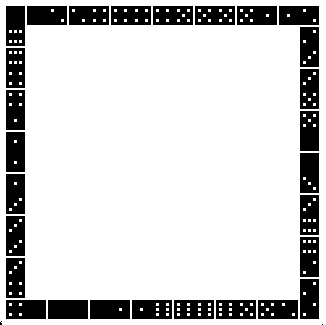You can easily make a square framework 15x15 from all dominos.

2nd and 3rd examples: Symmetrical figures with axis and centre point topYou should lay the crossings first. There you need 3 squares with the same number of points. Therefore you must use dominos with the same number of points also for another crossing, because there are 6*2 squares altogether.

4th example: A symmetrical figure with one axis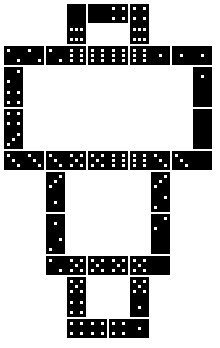5th example: Symmetrical figures with a centre point (Book 2 or book 3)
 ...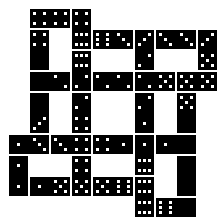... This is a well-known figure, which you can form with all the 28 dominos. You get more patterns, if you reflect the figure, or, this is more interesting, if you permutate the numbers of points on the dominos. You can replace the squares (0,1,2,3,4,5,6) by the squares (3,5,1,0,6,2,4) for example.

6th example: Variation of the third example
 ...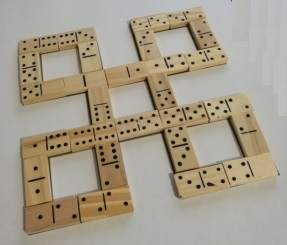Valerio Bianconi from Italy sent me a solution of a new figure.

 ...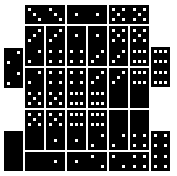... Quadrilles go back to the French mathematician  Edouard Lucas (1842-1891). Those are compact figures with all 28 dominos. 2x2squares with the same numbers of points are included in.  Here is an example.

Seven Squares  top

1st problem:You can lay seven square frames with all the 28 dominos. Dominos with the same number of points meet.

2nd problem:You can lay seven square frames with all the 28 dominos, so that the sums of the numbers of points are the same at all four sides (book 1).

The simple square frame 15x15 from the top also has the feature of the same sum at all four sides. The sum is (1+2+3+4+5+6)*8/4 = 44.

Making pairs   top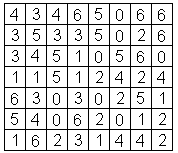You give a 7-8-matrix with the numbers 0 to 6, each number eight times. These are the squares, which the dominos have.  The problem is to assemble two squares lying underneath the other or side by side.
Tip: You should find the dominos with the same number ("doublets") first.

This is a solution.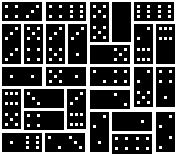You can invent this kind of puzzle yourself.

Dominos on the Internet top

Englisch

Dominoes

Teun Spaans
Domino Plaza

Wikipedia
Dominoes

Deutsch

Otto Janko
Domino-Puzzles

Wikipedia
Domino (Spiel)

Polnisch

Lamiglówki Mix
Domino

Russisch

Leonid Mochalov [PUZZLES of LEONID MOCHALOV]
DOMINO SOLITAIRE, Puzzles with Dominoes  (translated to English)

References   top
(1) Walter Sperling: Auf du und du mit Zahlen, Rüschlikon-Zürich 1955
(2) Pieter van Delft, Jack Botermans: Denkspiele der Welt, München 1980
(3) Karl-Heinz Koch: ...lege Spiele, Köln 1987 (dumont taschenbuch1480)
(4) Martin Gardner: Mathematischer Zirkus, Frankfurt a.M. 1988

Feedback: Email address on my main page